## Detailed Instructions for Setup of 'Spartan2 Lambda 02 Sensor' EFIS Data Port

Configure the analog data port using the EFIS setup screens. In most cases you will:
• Press the 'Menu' key;
• Select 'System Setup Menu';
• Select 'Analog Inputs Setup';
• Select the port the Spartan O2 sensor is attached to;
• Scroll down until you see these input fields: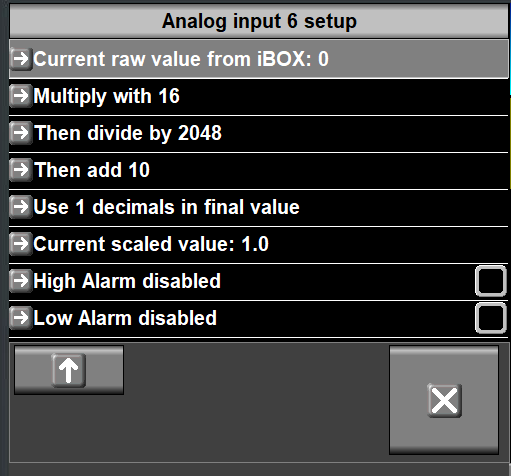• Fill in the table as follows:
 Multiply with 16 Then divide by 2048 Then add 10 Use 1 decimals in final value 1

# Troubleshooting

1. Check the analog port setup on the EFIS and verify that it matches the values shown above.
2. If possible, connect the output of the O2 sensor to a volt meter, perform ground engine tests with various fuel/air mixtures, and check the actual voltage. The sensor should read from 0 volts to 5 volts. An AFR of 13% should produce a voltage of 1.5 volts.
3. O2 sensors are sensitive to leaded fuels! If you use leaded fuel verify that the sensor is working correctly use the method recommended by the manufacturer.

# Conversion Formula

The formulas contain constants which are defined in these documents:
Step 1. Convert the raw value to volts. The constants used in this formula are defined in ref i at the top of page 7.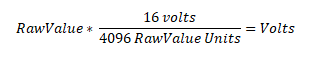Step 2. Convert the voltage to AFR in the range 0 to 10. The constants used in this formula are defined in ref ii.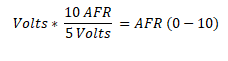Step 3. Add 10 to the 0-10 AFR range value to account for the fact that 0 Volts = 10 AFR. ref ii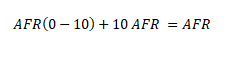Step 4. Refactor constants in the numerators and demominators.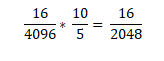The value 16 is used in the EFIS port configuration 'multiply by' field; 2048 is used in the 'divide by' field; and 10 (from step 3) is used in the 'then add' field.